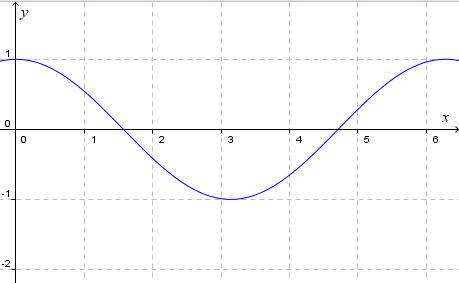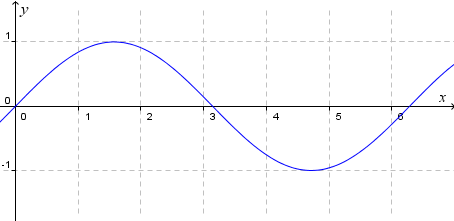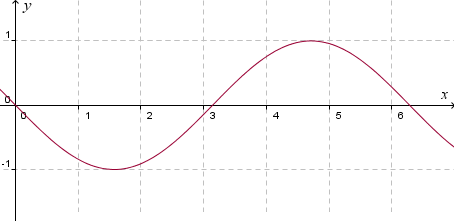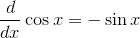Search IntMath
Close

# Explore the slope of the cos curve

By Murray Bourne, 26 Oct 2010

UPDATE: I've created a new interactive trigonometry derivatives applet to replace the out-of-date one that was on this page.

The background below is still worth reading!

## Background

This article is the second in a series. (Here's the first installment: Explore the slope of the sin curve, and the 3rd: Explore the slope of the tan curve.)

First, some reminders so you can better follow what is going on.

The slope of a curve means the slope of the tangent at a particular point. A tangent to a curve means the line that touches the curve at one point only. (See Tangents and Normals).

## Curve Reminders

#### Graph of cos x

Here is the curve y = cos x. The values of x are in radians and one complete cycle goes from 0 to (or around 6.28).y = cos x

#### Graph of sin x

The graph of y = sin x has the same shape as the cosine curve, but has been displaced (shifted) to the right by π/2 (or 90°).y = sin x

We now graph the negative of the above curve, that is, y = −sin x. To achieve this, we reflect the curve in the x-axis. This has the effect of multiplying each y-value in the curve by −1. Putting this another way, we "turn the graph upside-down", through the x-axis.y = −sin x

Now we are ready to investigate tne slope of the curve y = cos x using a GeoGebra-based JSXGraph interactive graph.

First, have a look at the graph below and observe the slope (m = -0.5) of the (red) tangent line at the point A is the same as the y-value of the point B (0.53, -0.5).

Then slowly drag the point A and observe the curve traced out by B. (The point B has the same x-value as point A, and its y-value is the same as the slope of the curve at point A).

Update: This applet has been replaced with a new one here:

Hopefully you can see that B traces out the curve −sin x. In other words, the slope of the graph y = cos x at any point (x,y) has value −sin x. Using calculus, we would write this as:See the first article in this series: Investigate the slope of y = sin x

In the next article, we'll examine the slope of y = tan x.

See more on the differentiation of sin, cos and tan curves. (This is in the calculus section of IntMath.)

### Comment Preview

HTML: You can use simple tags like <b>, <a href="...">, etc.

To enter math, you can can either:

1. Use simple calculator-like input in the following format (surround your math in backticks, or qq on tablet or phone):
a^2 = sqrt(b^2 + c^2)
(See more on ASCIIMath syntax); or
2. Use simple LaTeX in the following format. Surround your math with $$ and $$.
$$\int g dx = \sqrt{\frac{a}{b}}$$
(This is standard simple LaTeX.)

NOTE: You can mix both types of math entry in your comment.